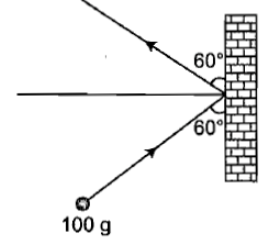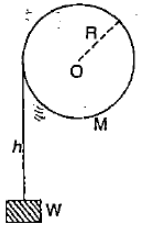A constant torque acting on a uniform circular wheel changes its angular momentum from ${\mathrm{A}}_{0}$ to  in 4s. The magnitude of this torque is

1. $\frac{3{\mathrm{A}}_{0}}{4}$

2.

3. ${\mathrm{A}}_{0}$

4.

Concept Questions :-

Angular momentum
High Yielding Test Series + Question Bank - NEET 2020

Difficulty Level:

Two discs are rotating about their axes, normal to the discs and passing through the centres of the discs. Disc D${}_{1}$ has 2 kg mass and 0.2 m radius and initial angular velocity of 50 rad s${}^{-1}$. Disc D${}_{2}$ has 4 kg mass, 0.1 m radius and initial angular velocity of 200 rad s${}^{-1}$. The two discs are brought in contact face to face, with their axes of rotation coincident. The final angular velocity (in rad.s${}^{-1}$) of the system is

1. 60

2. 100

3. 120

4. 40

Concept Questions :-

Angular momentum
High Yielding Test Series + Question Bank - NEET 2020

Difficulty Level:

A body rolls down an inclined plane without slipping. The fraction of total energy associated with its rotation will be

Where k is radius of gyration of the body about an axis passing through centre of mass and R is the radius of the body.

Concept Questions :-

Rolling motion
High Yielding Test Series + Question Bank - NEET 2020

Difficulty Level:

A mass of 100 g strikes the wall with speed 5 m/s at an angle as shown in figure and it rebounds with the same speed. If the contact time is $2×{10}^{-3}$ s, what is the force applied on the mass by the wall(1) N to right

(2) 250 N to right

(3) $250\sqrt{3}$ N to left

(4) 250 N to left

Concept Questions :-

Linear momentum
High Yielding Test Series + Question Bank - NEET 2020

Difficulty Level:

In the following figure, a weight W is attached to a string wrapped round a solid cylinder of mass M mounted on a frictionless horizontal axle at O.

If the weight starts from rest and falls a distance h, then its speed at that instant is(1) Proportional to R

(2) Proportional to $\frac{1}{R}$

(3) Proportional to $\frac{1}{{R}^{2}}$

(4) Independent of R

Concept Questions :-

Torque
High Yielding Test Series + Question Bank - NEET 2020

Difficulty Level:

A solid cylinder rolls down an inclined plane that has friction sufficient to prevent sliding. The ratio of rotational energy to total kinetic energy is

1.  $\frac{1}{2}$

2.  $\frac{1}{3}$

3.  $\frac{2}{3}$

4.  $\frac{3}{4}$

Concept Questions :-

Rolling motion
High Yielding Test Series + Question Bank - NEET 2020

Difficulty Level:

A swimmer while jumping into water from a height easily forms a loop in the air, if

1. He pulls his arms and legs in

2. He spreads his arms and legs

3. He keeps himself straight

4. None of the above

Concept Questions :-

Angular momentum
High Yielding Test Series + Question Bank - NEET 2020

Difficulty Level:

Assertion : A ladder is more apt to slip when you are high up on it than when you just begin to climb

Reason : At the high point on a ladder, the torque is large and on climbing up the torque is small

1. If both the assertion and the reason are true and the reason is a correct explanation of the assertion
2. If both the assertion and reason are true but the reason is not a correct explanation of the assertion
3. If the assertion is true but the reason is false
4. If both the assertion and reason are false

Concept Questions :-

Torque
High Yielding Test Series + Question Bank - NEET 2020

Difficulty Level:

An inclined plane makes an angle of $30°$ with the horizontal. A solid sphere rolling down this inclined plane from rest without slipping has a linear acceleration equal to

1.  $\frac{g}{3}$

2.  $\frac{5g}{7}$

3.  $\frac{2g}{3}$

4.  $\frac{5g}{14}$

Concept Questions :-

Rolling motion
High Yielding Test Series + Question Bank - NEET 2020

Difficulty Level:

Which of the following statements is correct?

1.   Kinetic energy and momentum both are conserved in all types of collisions

2.   Total kinetic energy is not conserved but momentum is conserved in inelastic COI-
lisions

3.  Momentum is conserved In elastic collisions but .not in inelastic collisions

4.  Total kinetic energy is conserved in inelastic collisions but momentum is not conserved in elastic collisions

Concept Questions :-

Linear momentum